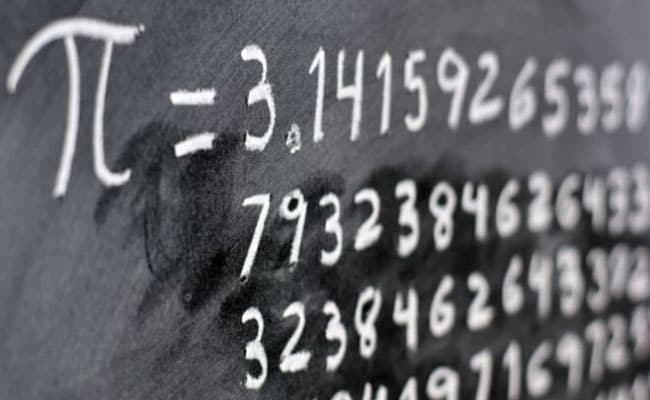# Pi Day 2022: Know 10 Surprisingly Interesting Facts About Pi

## Pi Day 2022: Celebrated on March 14, Pi Day celebrates the mathematical constant Pi, often depicted as its approximate decimal, 3.14.Pi is an infinitely long, irrational number and its exact value cannot be known.

Pi Day celebrates the mathematical constant Pi, and is significant for those who love mathematics and deal with this field of study. It is celebrated on March 14 and often depicted as the fraction 22/7 or as its approximate decimal, 3.14.

## To mark the day, here are 10 interesting facts about Pi:

1. Pi is an infinitely long, irrational number and its exact value cannot be known.

2. Since Pi's exact value cannot be known, we can never find the exact area or circumference of a circle.

3. Interestingly, legendary mathematician Albert Einstein's birth anniversary falls on Pi day.

4. Pi is a part of Egyptian mythology. It is being said that the Pyramids of Giza are built with the principles of Pi.

5. The value of the Pi was first calculated by Archimedes.

6. The Pi symbol was introduced by William Jones, a Welsh mathematician, in 1706.

7. In 1737, Leonhard Euler popularised the usage of the symbol.

8. Pi Day was celebrated for the first time in 1988 by American physicist Larry Shaw.

9. The value of Pi was determined till a record-breaking 22 trillion decimal places in 2017.

10. The calculation of the value of Pi can be used as a stress test for a computer processor.